# The Definition of a Limit

Choose one of the following topics:

While a table of numbers can certainly suggest that a limit has a certain value, it cannot definitely prove that the limit has that value. For instance, look at the function we looked at earlier.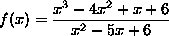The table of values we found earlier for x nearing 2 was:
 f(1.5) = 2.5 f(2.5) = 3.5 f(1.7) = 2.7 f(2.3) = 3.3 f(1.8) = 2.8 f(2.2) = 3.2 f(1.9) = 2.9 f(2.1) = 3.1 f(1.99) = 2.99 f(2.01) = 3.01 f(1.999) = 2.999 f(2.001) = 3.001

The values certainly look like they are approaching 3, but how do we know for certain? Perhaps they are approaching 3.000075 or 2.999996. What we need is a precise definition of a limit, which will tell us when we are exactly correct.

## Definition of Limit

 Let f be a function defined on some open interval that contains the number a, except possibly at a itself. We say the limit of f(x) as x approaches a is L, and we write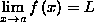if for every number> 0 there is a corresponding number> 0 such that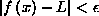whenever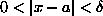.

Using our example function, f(x) is as above, a=2, and we think that L=3. Letbe any positive number. Then we can chooseto be equal to. In which case, if 0 < |x-2| <,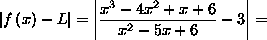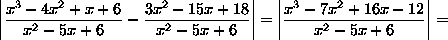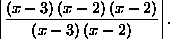We can cancel the (x-3) term from the top and bottom of the fraction, as well as one of the (x-2) terms from the top with the same on the bottom, to get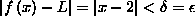.

So, since the definition fits exactly, we can state with certainty that the limit as x approaches 2 of f(x) is 3. Also, we can notice that if we used any value other than 3 for L, we wouldn't get the cancellation we did in the last step, so we would not have been able to fit the definition.

Below are the definitions of several related limit concepts.

## Left-Hand [or Right-Hand] Limit

 Let f be a function defined on some open interval (b,a) [or (a,b)]. We say the left-hand [or right-hand] limit of f(x) as x approaches a is L, (or the limit of f(x) as x approaches a from the left [or right] is L) and we write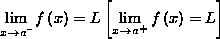if for every number> 0 there is a corresponding number> 0 such thatwhenever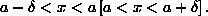## [Negative] Infinite Limit

 Let f be a function defined on some open interval that contains the number a, except possibly at a itself. We say the limit of f(x) as x approaches a is [negative] infinity, and we write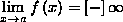if for every number N > 0 [N < 0] there is a corresponding number> 0 such that f(x) > N [f(x) < N] whenever.

## Limit at [Negative] Infinity

 Let f be a function defined on some open interval from a to infinity [from negative infinity to a]. Then we say the limit of f(x) as x approaches [negative] infinity is L, and we write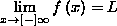if for every number> 0 there is a corresponding number N such thatwhenever x > N [x < N].

## [Negative] Infinite Limit at {Negative} Infinity

 Let f be a function defined on some open interval from a to infinity {from negative infinity to a}. We say the limit of f(x) as x approaches {negative} infinity is [negative] infinity, and we write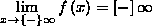if for every number M > 0 there is a corresponding number N such that f(x) > M [f(x) < M] whenever x > N {x < N}.

Similar definitions can be made for infinite-valued left- or right-hand limits.

Choose one of the following topics: